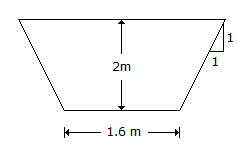# Civil Engineering - Waste Water Engineering - Discussion

### Discussion :: Waste Water Engineering - Section 3 (Q.No.36)

36.

For the open drain (N = 0.025) shown in the below figure, the discharge is[A]. 26.88 cumecs [B]. 27.88 cumecs [C]. 28.88 cumecs [D]. 29.88 cumecs.

Explanation:

No answer description available for this question.

 Gani said: (Dec 11, 2015) Where is slope?

 Shruthi said: (Feb 1, 2016) Please explain.

 Abdullah Talip said: (Jun 30, 2016) Assume slope = 0.01 in Hazen equation.

 Tayeb said: (Nov 22, 2016) AREA = 7.2 M2. 7.2/0.025 = 2.88.

 Harsha said: (Jul 29, 2017) Can anyone explain clearly?

 Shekhar Jana said: (Nov 11, 2017) Ratio of lateral axis should 1is 2, then area = 7.2 m2, 7.2/.025 = 2.88.

 Pravin said: (Feb 7, 2018) The Area is not considered in calculation. R is mean hydraulic radius i.e. area divided by wetted perimeter.

 Gosa Alemayehu said: (Oct 25, 2018) Please given the clear description for the answer.

 Anomie said: (Jan 8, 2019) Area =7.2, n=0.025, R=d/2=2/2=1, s=1. Use manning eqn to find vel. q = 28.88 cumecs.

 D H Unnadpotra said: (Jan 9, 2019) Area = 7.2 m2. Perimeter P=7.2 m. Therefore Hydraulic radius R = 1 m, n = 0.025. S = 1 and now when put these all values in manning's equation, V = 288 m/sec and Discharge Q = 39.82 cumecs. How it comes 28.88 cumecs? Please tell me.

 Kruthi said: (Jun 17, 2019) How to calculate the area? Please tell me.

 Haris Khan said: (Sep 5, 2019) A= D (B+(B+2nD)/2). Here D = 2 B = 1.6 n = 1( which is given as slope 1:1 we will take the base i.e horizontal) So, Area = 7.2.

 Dheeraj said: (Feb 8, 2020) It is very simple guys: The formula for Area of trapezoidal tank =Bd +sd^2. B - width. d - depth. S - slope(1vertical is to 1 horizontal i.e, =1). So, Area is (1.6 x 2)+(2^2)=7.2. So 7.2/.025 = 288.